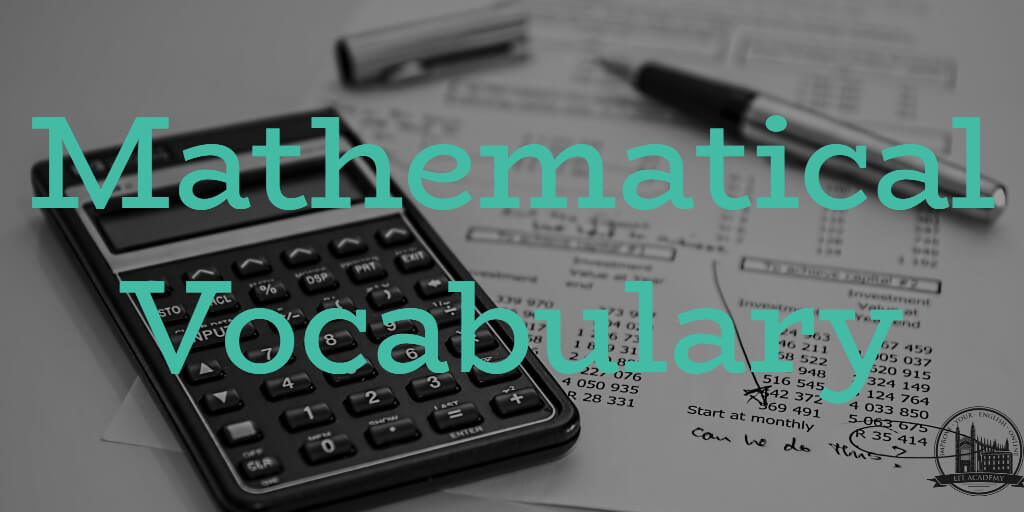# Mathematics Vocabulary in English

### Numbers

-187  :  minus one hundred and eighty seven
33,333  :  thirty-three thousand, three hundred and thirty-three
1,000,000  :  one million
2,000,000,000  :  two billion
9,000,000,000,000  :  nine trillion

Notes:

• always use a comma with numbers greater than 999.
• don’t say ‘3 millions’ – although you might hear people say things like ‘It cost millions and millions of dollars’.
• if you have an old dictionary you might read that there are two options for billion – that is no longer true.

54.25  : fifty-four point two five
110 : one to the power of ten
-5.5 x 1010 :  minus five point five times ten to the power of ten
8 x 10-3 :  eight times ten to the minus three

### Fractions

1 ½  :  one and a half
2 ⅓  :  two and a third
-3 ¼  :  minus three and a quarter
4 ⅕  :  four and a fifth
⅜  :  three-eighths
0.002  :  two thousandths

### Equations and Operations

3n + 4x  :  three n plus four x
1 – 2n  : one minus two n
3+3=6 :  three plus three equals six
18-8=10  :  eighteen minus eight equals ten
3/5=0.6  :  three divided by five equals zero point six
5!  =  five factorial

Notes:

• For 0.6 you can also say nought point six

### Greater and Less Than

x>y  :  x is greater than y
x≥y  :  x is greater than or equal to y
x<y  :  x is less than y
x≤y  :  x is less than or equal to y

Notes:

You can also say ‘x is smaller than y’

### Exponentials and Roots

22 :  two squared
43 :  four cubed
64 :  six to the power of four
810 :  eight to the power of ten
10-1 :  ten to the minus one
√3  :  the square root of three
3√32  :  the cube root of thirty two
5√64 :  the fifth root of sixty four

### Miscellaneous Maths Vocabulary

odd number   :  1,3,5,7, etc
even number  :  2,4,6, etc
denominator  : the number below the line
numerator  :  the number above the line
left bracket  :  [
right bracket  :  ]
curly bracket (AKA braces)  :  { }
exponent  :  how many times to use that number in a multiplication
factorial  :  the product of a number and all the numbers below it. (4! = 4 x 3 x 2 x 1)
infinity  :  ∞  :  a number greater than all the atoms of ice cream I’ve ever eaten
product  :  the answer when numbers are multiplied
ratio  :  the relationship between two numbers
integer  :  a whole number
rational number  :  a number that can be made by dividing two integers
irrational number  :  a real number that cannot be made by dividing two integers
prime  :  a number that is divisible only by itself and 1
coefficient  :  a number used to multiply a variable

Scroll to Top